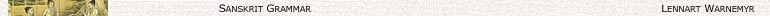Contents

# Augment (a)

A verbal S = [Pv] [K] A S- is ‘augmented’ (a S) in the following way:

 S = [Pv] K A S- > a S = [Pv] a K A S- (1) S = [Pv] A S- > a S = [Pv] VA S- (2)

Note:

In the principal Paradigms augmented forms are denoted by a non-bold ‘a’ before the rest of the stem.

Examples:

 [Pv] √ Impf/3/sg a ThS- a t bhū ‘become’ abhavat anusambhū ‘to be produced after’ anusamabhavat iṣ ‘desire’ aicchat udīṣ ‘rise, mount’ udaiṣat